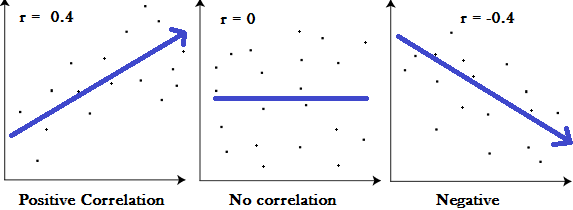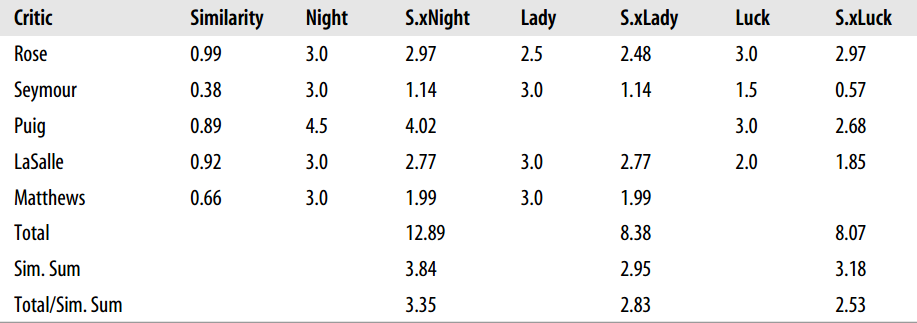《Programming Collective Intelligence》（中文名为《集体智慧编程》），是一本关于数据挖掘的书籍，每一章都会通过一个实际的例子来讲述某个机器学习算法，同时会涉及到数据的采集和处理等，是一本实践性很强的书籍。

1.寻找用户相似性的几种方法
2.基于用户相似性为用户推荐物品
3.寻找物品相似性的方法

寻找用户相似性的几种方法

皮尔逊相关系数

$$sim = \frac{\sum_{c \in I_{ij}}(R_{i,c} - \overline{R_i})(R_{j,c} - \overline{R_j})}{\sqrt{\sum_{c \in I_{ij}} (R_{i,c} - \overline{R_i})^2} \sqrt{\sum_{c \in I_{ij}} (R_{j,c} - \overline{R_j})^2} }$$

$I_{ij}$ 用户 $i$ 和用户 $j$ 的公共评分集，也就是两者都有评分的物品的集合
$R_{i,c}$ 用户 $i$ 对物品c的评分
$R_{j,c}$ 用户 $j$ 对物品c的评分
$\overline {R_i}$ 用户 $i$ 对 $I_{ij}$ 中物品评分的均值
$\overline {R_j}$ 用户 $j$ 对 $I_{ij}$ 中物品评分的均值High correlation 0.5~1.0 or -0.5~ -1.0
Medium correlation 0.3 ~ 0.5 or -0.3~ -0.5
Low correlation 0.1~0.3 or -0.1~ -0.3

修正的余弦相似性

$$sim = \frac{\sum_{c \in I_{ij}}(R_{i,c} - \overline{R_i})(R_{j,c} - \overline{R_j})}{\sqrt{\sum_{c \in I_i} (R_{i,c} - \overline{R_i})^2} \sqrt{\sum_{c \in I_j} (R_{j,c} - \overline{R_j})^2} }$$

$I_{ij}$ 用户 $i$ 和用户 $j$ 的公共评分集，也就是均被两者评分的物品的集合
$I_i$ 被用户 $i$ 评分了的物品的集合
$I_j$ 被用户 $j$ 评分了的物品的集合
$R_{i,c}$ 用户 $i$ 对物品c的评分
$R_{j,c}$ 用户 $j$ 对物品c的评分
$\overline {R_i}$ 用户 $i$ 所有评分的均值
$\overline {R_j}$ 用户 $j$ 所有评分的均值

$$sim = \frac{\sum_{c \in I_{ij}}(R_{i,c} - \overline{R_i})(R_{j,c} - \overline{R_j})}{\sqrt{\sum_{c \in I_{ij}} (R_{i,c} - \overline{R_i})^2} \sqrt{\sum_{c \in I_{ij}} (R_{j,c} - \overline{R_j})^2} }$$

为用户推荐物品